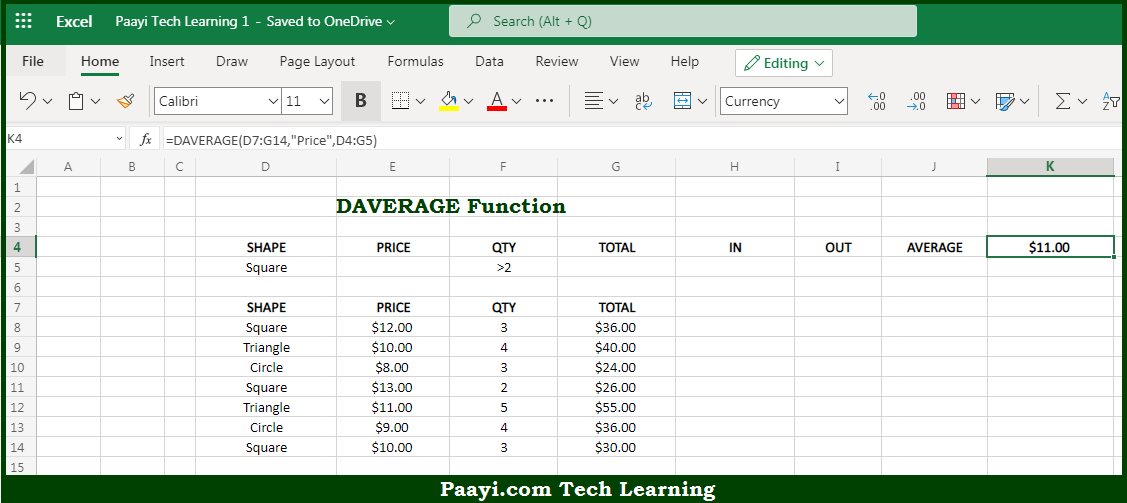# Learn How to Use Microsoft Excel DAVERAGE Function

Written by | 0 Comments | 648 Views

In this article, you will learn how to use the Microsoft Excel DAVERAGE function and its prime function in Microsoft Excel. You will also get to know the Microsoft Excel DAVERAGE function return value and syntax with the help of some examples.

Microsoft Excel DAVERAGE Function

The main purpose of the Microsoft Excel DAVERAGE function is to get the average from matching records. That implies, with the help of DAVERAGE function you can able to return the average in a given field for records that match criteria. So, with the help of DAVERAGE function, you can able to get the average from matching records.

Return Value of DAVERAGE Function

The return value will be the average value in the given field.

Syntax of DAVERAGE Function

=DAVERAGE(database, field, criteria)

Where the arguments:

• database: This is the database range including headers.
• field: This is a field name or index to count.
• criteria: This is the criteria range including headers.

## How to Use Microsoft Excel DAVERAGE Function?So we know that Microsoft Excel DAVERAGE function you can able to get the average from matching records. That implies, with the help of the DAVERAGE function you can able to return the average in a given field for records that match criteria. So, with the help of the DAVERAGE function, you can able to get the average from matching records.